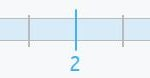Smartick is a fun way to learn math!# I want to learn about: Fractions

Content about fractions in elementary mathematics. Exercises, tutorials, problems, and teaching resources for fractions in elementary. Here you will find posts about fractions to make learning mathematics easier and more fun.

Aug31

## Direct and Inverse Proportionality ProblemsIn today’s post, we are going to work on proportionality by looking at some examples of proportionality problems. Before we begin, you can take a look at other exercises with proportional numbers where we review the concepts of reason and proportionality constant. Direct Proportionality and Inverse Proportionality Proportional magnitudes can be directly proportional or inversely […]

May05

## Half, Third, Fourth, Fifth in Math: Definition & CalculationIn this post, we are going to learn how to calculate a half, third, fourth, and fifth. These expressions are not just used for math problems, but also in daily life! Do you know what they are? Do you know how to calculate them? You are going to see how easy it is to calculate […]

Apr20

## Adding Fractions with Different Denominators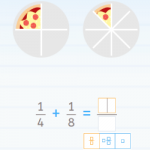We are going to begin this post about adding fractions with three very simple questions: 1. If you had one apple and were given two apples, how many apples do you have now? 2. If you had one apple and were given half an apple, how many apples do you have now? 3. If you […]

Mar16

## Equivalent Fractions on a Number Line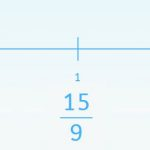Today we are going to learn how to solve activities related to the representation of fractions on a number line and the amplification and simplification of fractions. To do this we need to use equivalent fractions. It is easy to represent a fraction on a number line when it is divided into the same number […]

Nov18

## What Is a Fraction? Learn Everything There Is to Know!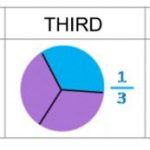On the Smartick blog we have dedicated many entries to explaining what a fraction is, what types there are, how to do addition, subtraction, multiplication and division with them, equivalent fractions and much more! In today’s post, we are going to organize these entries to make it easier for you to find the content you […]

Jul29

## Do You Know What an Equivalent Fraction Is?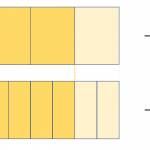Do you know what an equivalent fraction is? We have written about equivalent fractions many times on the blog: Equivalent Fractions Practice with Examples of Equivalent Fractions Examples of Equivalent Fractions Today, we are going to start by investigating the etymology, or meaning, of the word ”equivalent.” Let’s split the word in two: equi – […]

Apr29

## Multiplication of Fractions with an Area Model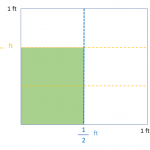In today’s post, we are going to see how to use an area model to solve a multiplication problem with fractions. This methodological strategy is very effective to show our students an operation that is very complicated to visualize. Teachers usually make an effort to support their explanations with manipulatives and visuals, because if they […]

Mar04

## Problems with Fractions and How to Solve ThemIn this post, we are going to give you some guidelines and advice for confronting problems with fractions. Problems with fractions, in no time! Fortywinklesburg is an imaginary kingdom where all of its inhabitants have a peculiar habit: they sleep for days without waking up. The Winklean’s, as they are called, sleep for so long […]

Feb11

## Rule of Three for Calculating PercentagesIn earlier posts, we have learned what a percent is and how to calculate it. Today we are going to use the rule of three to solve different types of problems related to percentages. Rule of three to calculate the percentage of a number For example, we want to calculate 30% of 360. 30% means […]

Jan07

## What Do Double and Half Mean?In today’s post, we are going to talk about the concepts double and half. First, I will explain what each term means and then we will see a strange situation with them. Double and half explain a type of relationship that exists between two numbers or quantities. Double of a quantity The double of a […]

Nov19

## How to Add 3 Fractions with Different Denominators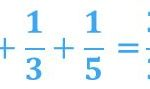On the Smartick blog, we have written a lot about fractions and the different operations we can do with them. In today’s blog post you can find much more information! Until now, we had not written about operations with more than 2 fractions. In this post, you are going to learn to add 3 fractions […]